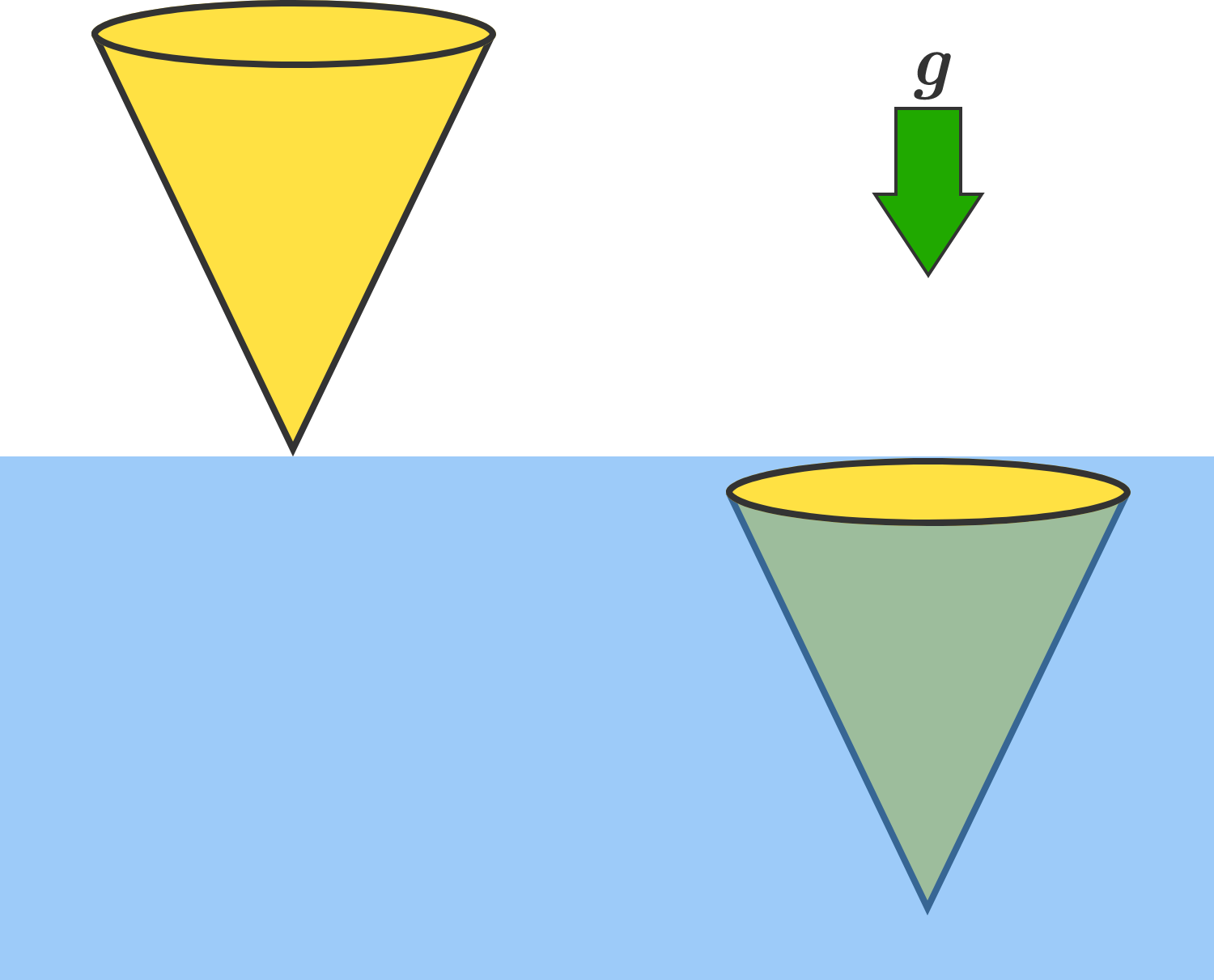# Diving ConeA solid right circular cone of uniform mass density is initially at rest above a body of water, so that its vertex is just touching the water's surface with its axis of symmetry along the vertical.

Now, the cone falls into the water, and has zero speed at the instant it becomes fully submerged. What is the ratio of the density of the cone to the density of the water? Submit your answer to 2 decimal places.


Details and Assumptions:

• There is an ambient downward gravitational field.
• Assume that the buoyant force is the only force exerted by the water on the cone.
×